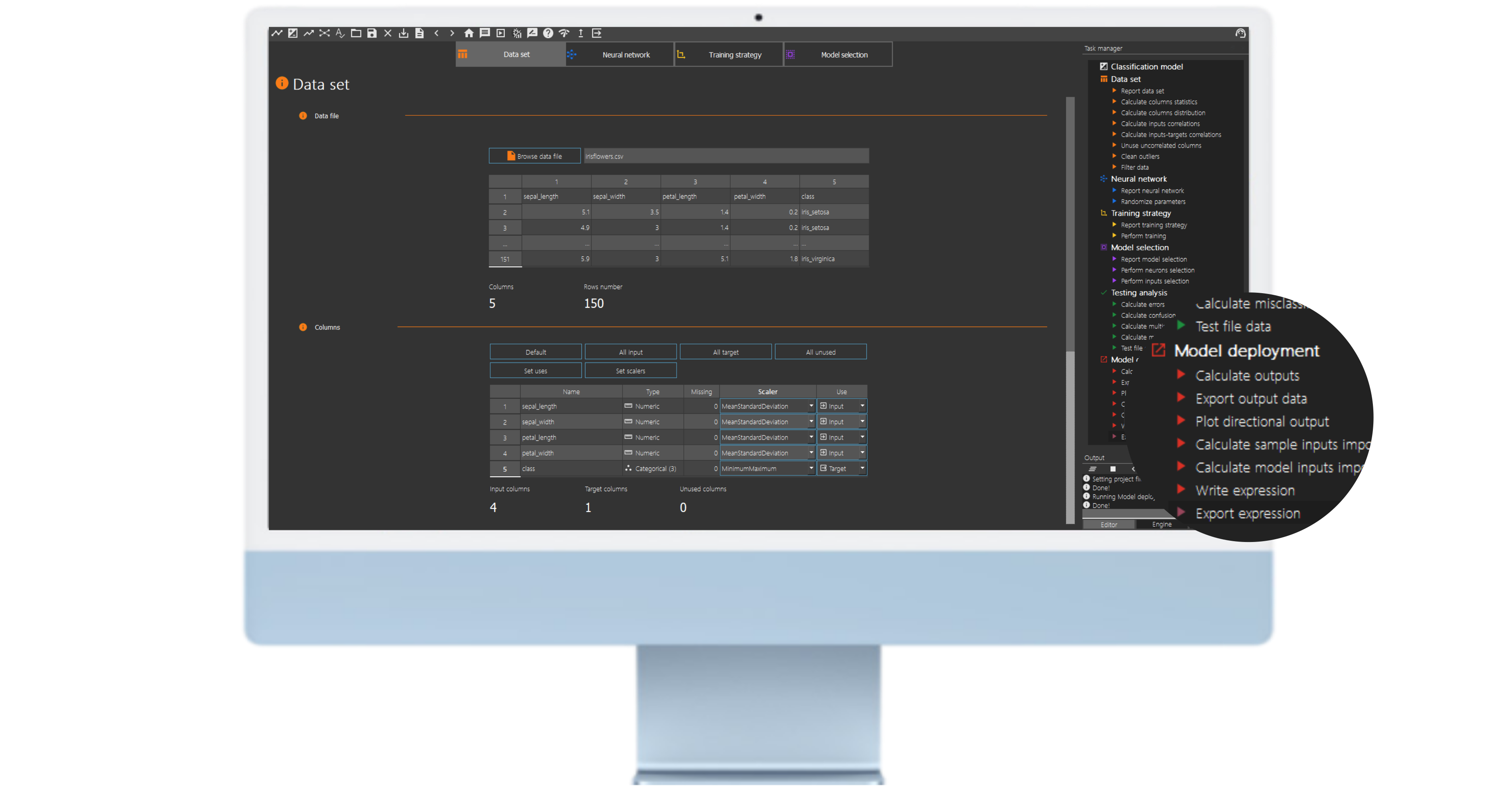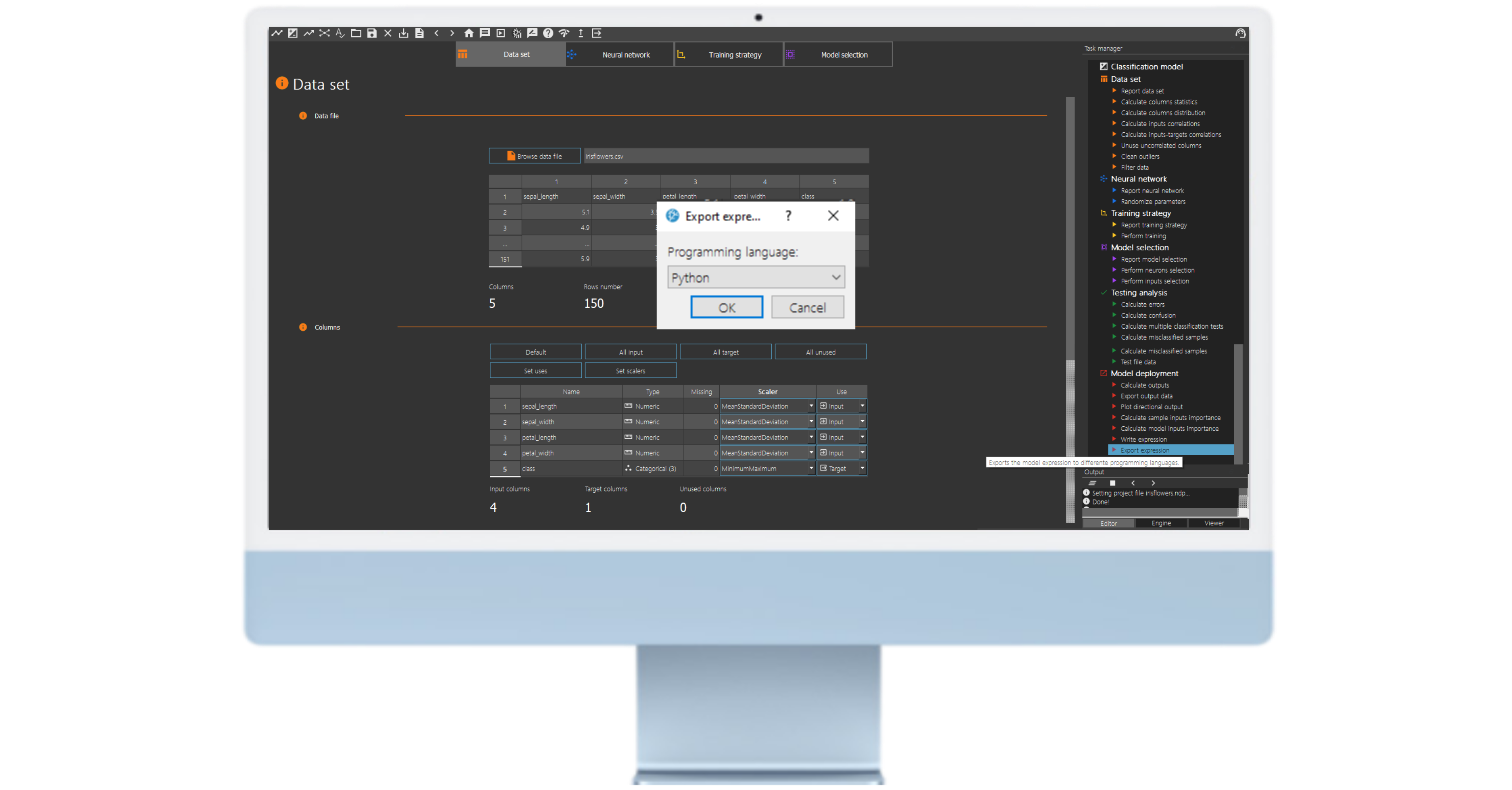## 1. Introduction

Neural Designer is a powerful tool for building and analyzing neural network models. However, when working with these models, it is crucial to have access to the underlying mathematical expressions that govern their behavior. Fortunately, Neural Designer provides several options for working with these expressions.

The predictive model takes the form of a function of the outputs concerning the inputs.

We can export the mathematical expression the model represents to different programming languages.

In this tutorial, we will explain how to export the mathematical expression of our model in the Python programming language.

## 2. How to export the mathematical expression?

Once we have trained the model and performed the testing analysis, the neural network can predict outputs for inputs it has never seen. This process is called model deployment.

We obtain the mathematical expression of the trained neural network.

We can implement this expression in any programming language to obtain the output for our input.

### Example: The mathematical expression of the iris flower classification model

Classification of iris flowers is the best-known example of machine learning.

The aim is to classify iris flowers among three species (Setosa, Versicolor, or Virginica) from the measurements of sepals’ and petals’ length and width.

The central goal is to design a model that makes proper classifications for new flowers. In other words, one which exhibits good generalization.

After training and testing the model, we move on to the model deployment process.One such option is “write expression”, which allows users to view the mathematical expression represented by the neural network of the model. By clicking on this option, Neural Designer will display the model’s function to predict outputs based on inputs. This information can be crucial for understanding how the model works and making modifications to improve its performance.

Another option available to users is “export expression”. Users can export the mathematical expression the model represents in several programming languages by selecting this option. This can be particularly useful for incorporating the model into other software programs or integrating it into larger systems.To export the mathematical expression in Python code, choose this option from the drop-down menu and save it in the model directory.

## 3. Using a Pre-Trained Neural Network Model in Python

Exporting the mathematical expression of a pre-trained neural network in Python allows us to use the trained model to make predictions on new data sets without the need to retrain the network from scratch.

Example: Using the Pre-Trained iris flower classification model in Python

The next listing is iris flower classification model neural network in the Python programming language

Artificial Intelligence Techniques SLartelnics@artelnics.com

Your model has been exported to this python file. You can manage it with the ‘NeuralNetwork’ class.



Example:

model = NeuralNetwork()

sample = [input_1, input_2, input_3, input_4, ...]

outputs = model.calculate_output(sample)


Inputs Names:
0) sepal_length
1) sepal_width
2) petal_length
3) petal_width

You can predict a batch of samples using the calculate_batch_output method. IMPORTANT: input batch must be type.

Example_1:model = NeuralNetwork()input_batch = np.array([[1, 2], [4, 5]])outputs = model.calculate_batch_output(input_batch)Example_2:input_batch = pd.DataFrame( {'col1': [1, 2], 'col2': [3, 4]})

outputs = model.calculate_batch_output(input_batch.values)

'''

import math

import numpy as np

class NeuralNetwork:

def __init__(self):self.inputs_number = 4

def calculate_outputs(self, inputs):

sepal_length = inputssepal_width = inputspetal_length = inputspetal_width = inputs

scaled_sepal_length = (sepal_length-5.843329906)/0.8280659914

scaled_sepal_width = (sepal_width-3.053999901)/0.4335939884scaled_petal_length = (petal_length-3.758670092)/1.764420033scaled_petal_width = (petal_width-1.19867003)/0.7631610036

perceptron_layer_1_output_0 = np.tanh( -0.183118 + (scaled_sepal_length*0.134644) + (scaled_sepal_width*0.149121) + (scaled_petal_length*-0.102136) + (scaled_petal_width*-0.0227783) )

perceptron_layer_1_output_1 = np.tanh( -0.0550171 + (scaled_sepal_length*-0.144019) + (scaled_sepal_width*0.188538) + (scaled_petal_length*0.126428) + (scaled_petal_width*-0.0199585) )perceptron_layer_1_output_2 = np.tanh( -0.131689 + (scaled_sepal_length*0.195227) + (scaled_sepal_width*0.0878418) + (scaled_petal_length*0.196912) + (scaled_petal_width*0.104858) )

probabilistic_layer_combinations_0 = -0.11217 -0.10332*perceptron_layer_1_output_0 +0.0966187*perceptron_layer_1_output_1 -0.0124268*perceptron_layer_1_output_2

probabilistic_layer_combinations_1 = -0.0184937 +0.012561*perceptron_layer_1_output_0 -0.0662964*perceptron_layer_1_output_1 -0.0870483*perceptron_layer_1_output_2probabilistic_layer_combinations_2 = 0.0834106 +0.153918*perceptron_layer_1_output_0 +0.0744019*perceptron_layer_1_output_1 -0.15564*perceptron_layer_1_output_2

sum = np.exp(probabilistic_layer_combinations_0) + np.exp(probabilistic_layer_combinations_1) + np.exp(probabilistic_layer_combinations_2)

iris_setosa = np.exp(probabilistic_layer_combinations_0)/sum

iris_versicolor = np.exp(probabilistic_layer_combinations_1)/sumiris_virginica = np.exp(probabilistic_layer_combinations_2)/sum

out = [None]*3

out = iris_setosaout = iris_versicolorout = iris_virginica

return out

def calculate_batch_output(self, input_batch):

output_batch = [None]*input_batch.shape

for i in range(input_batch.shape):

inputs = list(input_batch[i])

output = self.calculate_outputs(inputs)

output_batch[i] = output

return output_batch


The code starts with an explanatory text string in triple quotes describing how to use the neural network. It then defines a Python class called NeuralNetwork. The class has two methods: calculate_outputs and calculate_batch_output. The first method calculates the neural network’s output for a single set of inputs, while the second method calculates the neural network’s output for a batch of input sets.

The calculate_outputs method lists four input values (sepal length, sepal width, petal length, and petal width) and returns a list of three output values (probabilities for each iris species). First, the method uses the predefined weights for each perceptron in the hidden layer to calculate the hidden layer output. Then, it uses the predefined weights for the output layer to calculate the probability distribution for each iris species.

The calculate_batch_output method takes an input_batch input matrix containing a set of inputs for various iris flower examples
and returns an output_batch output matrix containing the probability distribution for each iris flower example.

To use the model in your Python project, you should follow these steps:

1. Save the model.py file in the same folder as your main Python file (the file you run).

2. Import the NeuralNetwork class from the model.py file in your main Python file using the following code:

from mode import NeuralNetwork

3. Create an instance of the NeuralNetwork model in your main Python file:

model = NeuralNetwork()

4. To predict the iris species for a single input set, you should pass the inputs as a list to the calculate_outputs() function of the model instance:

inputs = [5.1, 3.5, 1.4, 0.2] # example inputsoutputs = model.calculate_outputs(inputs)print(outputs)

This will return a list of three values representing the predicted probabilities for each iris species: [0.9818439478701074, 0.01815605212989281, 4.239074497248452e-16].

5. To predict the iris species for a batch of inputs, you should pass the inputs as a numpy array to the calculate_batch_output() function of the model instance:

import numpy as np

input_batch = np.array([[5.1, 3.5, 1.4, 0.2], [4.9, 3.0, 1.4, 0.2]]) # example inputs
outputs = model.calculate_batch_output(input_batch)
print(outputs)

This will return a two rows and three columns matrix representing the predicted probabilities for each iris species for each input set: [[0.98184395, 0.01815605, 4.23907450e-16], [0.97104675, 0.02895325, 5.88727745e-17]].

## 5. Conclusions

The mathematical expression of a pre-trained neural network exported in different programming codes enables us to use the trained model to make predictions on new data sets without retraining the network from scratch.

Exporting the pre-trained mathematical expression of a neural network allows us to leverage the knowledge and generalization capability of the model in different applications and projects. This is a fundamental step to take full advantage of the potential of artificial intelligence and machine learning in Python.